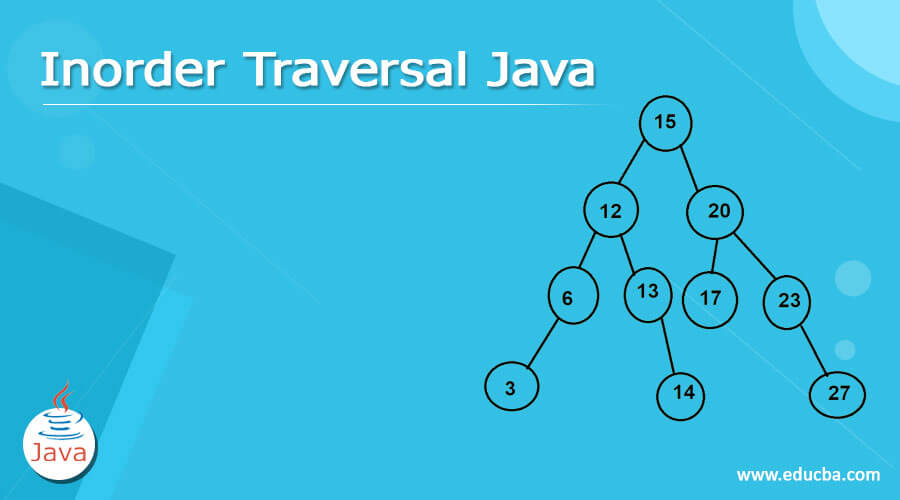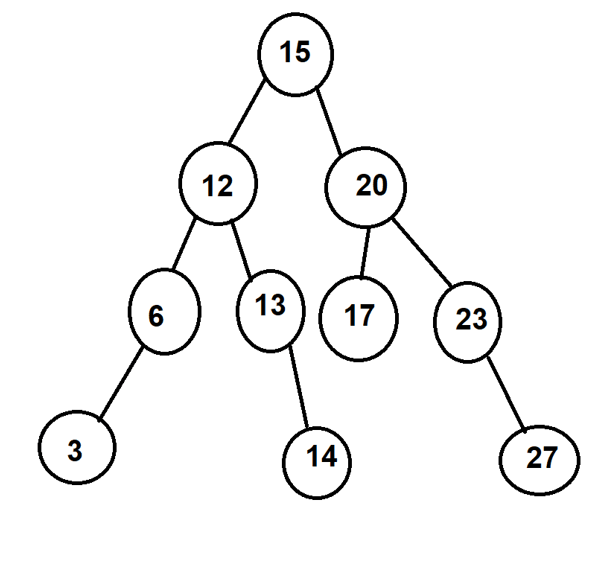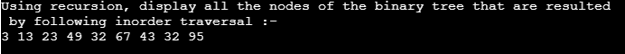# Inorder Traversal Java | Working of Inorder Traversal | Example

21## Introduction to Inorder Traversal

Inorder traversal of a tree is the way of visiting each of the nodes of the tree in the order that the leftmost node is visited first then the root and lastly the rightmost node. Traversing is the way in which we visit each of the elements of values of the given data structure. Each of the linear data structures has only one way in which its elements can be traversed. Linear data structures are arrays, stacks, queues, and linked lists. But in the case of a non-linear data structure like tree, there are multiple possible ways and orders in which the nodes can be visited. There are three basic orders inorder, preorder, and postorder traversal methods.

In this article, we will study the in-order traversal method and how it works in the binary tree. We will also see one of the examples of how in order traversal can be implemented using the C programming language.

### Working of Inorder Traversal

The algorithm or steps used while traversing a tree using inorder traversal are as listed below –
• The first thing to do is to traverse all the nodes of the left sub tree in the manner where the first left node is visited then the main and then the right node.
• Now, visit the root node of the tree.
• It’s time to visit the right subtree after left subtree traversing and root visiting.

Consider a tree in which the nodes are as shown in the figure –In the above given example of the tree, the traversing will first start from the left most node or leaf of the plant which is 3, and then we will traverse its main immediate parent which is 6. After that, we will go for searching its right child, but, as we can see there’s no right child of the 6 nodes, hence, will now visit the immediate parent of the 6 node which is 12, and in this way will continue our journey of traversing. Finally, the resultant order of traversal will be as shown below –
3 -> 6 -> 12 -> 13 -> 14 -> 15 -> 20 -> 17 -> 23 -> 27

Applications of inorder traversal-

The inorder traversal is mostly used in binary search trees. The reversed algorithm of inorder traversal is used to get non-increasing order of node values. In case if we want the nodes to be retrieved in the non-decreasing format then there is no need to use a variation of inorder traversal. We can make use of the same inorder traversal method discussed above to get non-decreasing values of the binary tree.

Implementation of Inorder traversal using Java programming language-

For understanding the flow of the inorder traversal and its implementation in the Java programming language, you need to be aware of java methods and classes and object concepts. The following program uses a recursive call to the method of inorder traversal firstly for the left side subtree after that visiting the root node and then for the right side subtree. While visiting each of the nodes in the traversal the program makes sure to print the value of the same node visited. Hence, we can see in the output, how all the nodes are visited and in which order they are visited.
Program –

```import java.util.Stack; /* * Implementation of inorder traversal of the binary tree. * Inorder traversal involves travelling to he left most leaf or node of the * tree and then the root node of the tree. The last node to be traversed is * the rightmost node or leaf of the binary tree. * * input: * 43 * / * 23 32 * / * 13 32 95 * / / * 3 49 67 * * Resulting output: 3 13 23 32 43 32 95 49 67 */ public class Main { public static void main(String[] args) throws Exception { // Firstly, we will create the binary tree as displayed in the comments BinaryTreeToTraverse bt = BinaryTreeToTraverse.create(); // With the help of recursion that means calling the same function again and again, // we will do inorder traversal of the binary tree System.out .println("Using recursion, display all the nodes of the binary tree that are resultedn by following inorder traversal :- "); bt.inOrderTraversal(); } } class BinaryTreeToTraverse { static class binaryTreeNode { String data; binaryTreeNode leftNode, rightNode; binaryTreeNode(String value) { this.data = value; leftNode = rightNode = null; } } // Root node of the considered binary tree binaryTreeNode rootNode; /** * Use the inorder algorithm for traversing through the nodes in binary tree */ public void inOrderTraversal() { inOrderTraversal(rootNode); } private void inOrderTraversal(binaryTreeNode node) { if (node == null) { return; } inOrderTraversal(node.leftNode); System.out.printf("%s ", node.data); inOrderTraversal(node.rightNode); } /** * Consider the sample data to test the order in which the nodes are traversed in Java program * * @return a sample binary binaryTree for testing */ public static BinaryTreeToTraverse create() { BinaryTreeToTraverse binaryTree = new BinaryTreeToTraverse(); binaryTreeNode rootNode = new binaryTreeNode("43"); binaryTree.rootNode = rootNode; binaryTree.rootNode.leftNode = new binaryTreeNode("23"); binaryTree.rootNode.leftNode.leftNode = new binaryTreeNode("13"); binaryTree.rootNode.leftNode.leftNode.leftNode = new binaryTreeNode("3"); binaryTree.rootNode.leftNode.rightNode = new binaryTreeNode("32"); binaryTree.rootNode.rightNode = new binaryTreeNode("32"); binaryTree.rootNode.rightNode.rightNode = new binaryTreeNode("95"); binaryTree.rootNode.leftNode.rightNode.leftNode = new binaryTreeNode("49"); binaryTree.rootNode.leftNode.rightNode.rightNode = new binaryTreeNode("67"); return binaryTree; } }```

Output:The output of the execution of the above program is as shown below:

### Conclusion

The inorder traversal is one of the depth-first traversing methods in which all the nodes are traversed in the manner where firstly the left node is traversed and then the root and then the right subtree is traversed. The leftmost leaf of the tree is the first node to be visited while the rightmost leaf is the last one to be traversed in the inorder traversal. The inorder traversing method is extensively used in binary search trees for getting non-decreasing or non-increasing order of values. The java implementation can be either done in recursive format or iterative format. The recursion method is used here for implementation where the same method is called again and again for implementing inorder traversal.

### Recommended Articles

This is a guide to Inorder Traversal Java. Here we discuss Introduction, Working of Inorder Traversal, example with code implementation. You may also have a look at the following articles to learn more –

The post Inorder Traversal Java appeared first on EDUCBA.

Source linkFounder OF Postintrend.com started my blogging journey in 2015 In This Duration I learned more about blogging, SEO, SEM, Affiliate marketing and more kinds of stuff on the Internet. I love to share my information with everyone.